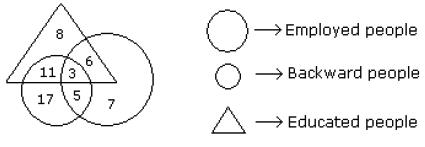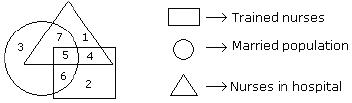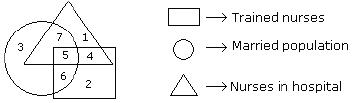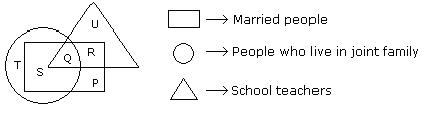Courses

# Olympiad Test: Venn Diagrams - 2

## 20 Questions MCQ Test Mathematics Olympiad Class 7 | Olympiad Test: Venn Diagrams - 2

Description
This mock test of Olympiad Test: Venn Diagrams - 2 for Class 7 helps you for every Class 7 entrance exam. This contains 20 Multiple Choice Questions for Class 7 Olympiad Test: Venn Diagrams - 2 (mcq) to study with solutions a complete question bank. The solved questions answers in this Olympiad Test: Venn Diagrams - 2 quiz give you a good mix of easy questions and tough questions. Class 7 students definitely take this Olympiad Test: Venn Diagrams - 2 exercise for a better result in the exam. You can find other Olympiad Test: Venn Diagrams - 2 extra questions, long questions & short questions for Class 7 on EduRev as well by searching above.
QUESTION: 1

### Direction: Each of these questions given below contains three elements. These elements may or may not have some inter linkage. Each group of elements may fit into one of these diagrams at (a), (b), (c), (d). You have to indicate the group of elements which correctly fits into the diagrams. Q. Which of the following diagrams indicates the best relation among Class, Blackboard and School?

Solution:

Blackboard is in class and class is in the school.

QUESTION: 2

### Direction: Each of these questions given below contains three elements. These elements may or may not have some inter linkage. Each group of elements may fit into one of these diagrams at (a), (b), (c), (d). You have to indicate the group of elements which correctly fits into the diagrams. Q. Which of the following diagrams indicates the best relation among Rabi-Crop, Paddy and Wheat?

Solution:

Wheat and Paddy are different from each other but Wheat is the Rabi-Crop.

QUESTION: 3

### Direction: Each of these questions given below contains three elements. These elements may or may not have some inter linkage. Each group of elements may fit into one of these diagrams at (a), (b), (c), (d). You have to indicate the group of elements which correctly fits into the diagrams. Q. Which of the following diagrams indicates the best relation among Hospital, Nurse and Patient?

Solution:

Hospital consists of nurse and patient but nurse and patient are of two different nature.

QUESTION: 4

Direction: Each of these questions given below contains three elements. These elements may or may not have some inter linkage. Each group of elements may fit into one of these diagrams at (a), (b), (c), (d). You have to indicate the group of elements which correctly fits into the diagrams.
Q. Which of the following diagrams indicates the best relation among Mercury, Zinc and Metal?

Solution:

Mercury and Zinc both are different from each other but belong to metal.

QUESTION: 5

Direction: Each of these questions given below contains three elements. These elements may or may not have some inter linkage. Each group of elements may fit into one of these diagrams at (a), (b), (c), (d). You have to indicate the group of elements which correctly fits into the diagrams.
Q. Which of the following diagrams indicates the best relation among Teacher, Writer and Musician?

Solution:

A teacher may or may not be a writer and musician. Similarly a musician may or may not be a teacher and writer and so a writer may or may not be a teacher and musician.

QUESTION: 6

Direction: Each of these questions given below contains three elements. These elements may or may not have some inter linkage. Each group of elements may fit into one of these diagrams at (a), (b), (c), (d). You have to indicate the group of elements which correctly fits into the diagrams.
Q. Which of the following diagrams indicates the best relation among Iron, Lead and Nitrogen?

Solution:

All the three elements are different from each other.

QUESTION: 7

Direction: Each of these questions given below contains three elements. These elements may or may not have some inter linkage. Each group of elements may fit into one of these diagrams at (a), (b), (c), (d). You have to indicate the group of elements which correctly fits into the diagrams.
Q. Which of the following diagrams indicates the best relation among Examination, Questions and Practice?

Solution:

Some questions are asked in examination and some in practice but examination and practice are different from each other.

QUESTION: 8

Direction: Each of these questions given below contains three elements. These elements may or may not have some inter linkage. Each group of elements may fit into one of these diagrams at (a), (b), (c), (d). You have to indicate the group of elements which correctly fits into the diagrams.
Q. Which of the following diagrams indicates the best relation among Bulb, Lamp and Light?

Solution:

Bulb and Lamp are different from each other but light is obtained from both.

QUESTION: 9

Direction: Each of these questions given below contains three elements. These elements may or may not have some inter linkage. Each group of elements may fit into one of these diagrams at (a), (b), (c), (d). You have to indicate the group of elements which correctly fits into the diagrams.
Q. Which of the following diagrams indicates the best relation among Lion, Dog and Snake?

Solution:

All the three are different from each other.

QUESTION: 10

Direction: Each of these questions given below contains three elements. These elements may or may not have some inter linkage. Each group of elements may fit into one of these diagrams at (a), (b), (c), (d). You have to indicate the group of elements which correctly fits into the diagrams.
Q. Which of the following diagrams indicates the best relation among Moon, Sun and Earth?

Solution:

All the three are different from each other.

QUESTION: 11

Direction: Study the following figure and answer the questions given below.Q. How many educated people are employed?

Solution:

Number of educated people who are employed = 3 + 6 = 9.

QUESTION: 12

Direction: Study the following figure and answer the questions given below.Q. How many backward people are educated?

Solution:

Number of backward people are who are educated = 11 + 3 = 14.

QUESTION: 13

Direction: Study the following figure and answer the questions given below.Q. How many backward uneducated people are employed?

Solution:

Number of backward uneducated people who are employed is 5.

QUESTION: 14

Direction: Study the following figure and answer the questions given below.Q. How many backward people are not educated?

Solution:

Number of backward people who are not educated = 17 + 5 = 22.

QUESTION: 15

Direction: Study the following figure and answer the questions given below.Q. If the hospital management requires only married trained nurses for operation theatre, which number of diagram should be chosen by it?

Solution:
QUESTION: 16

Direction: Study the following figure and answer the questions given below.Q. By which number, married but untrained nurses in the hospital are represented?

Solution:
QUESTION: 17

Direction: Study the following figure and answer the questions given below.Q. By which numbers trained nurses are represented?

Solution:
QUESTION: 18

Direction: Study the following figure and answer the questions given below.Q. What is represented by the number 7?

Solution:
QUESTION: 19

Direction: Study the following figure and answer the questions given below.Q. By which letter, the married teachers who live in joint family are represented?

Solution:
QUESTION: 20

Direction: Study the following figure and answer the questions given below.Q. By which letter, the married people who live in joint family but not are school teachers are represented?

Solution: